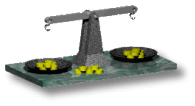# dotSphinx# Find The Forged Coin

Weigh the coins, and find out which one is forged

## Solution

This is the solution to the Find The Forged Coin game. There are 12 coins. Let's number them, from 1 to 12. Put coins 1-4 to the left, and coins 5-8 right. The result can be one of the following:
• Balance tends to the left:
This means that either one of 1-4 is too heavy, or one of 5-8 is too light. So, put coins 1, 2, 5 and 6 to the left and coins 3, 7, 9 and 10 to the right.
• Balance tends to the left:
So, either coin 1/2 is too heavy, or coin 7 is too light. Let's weigh coin 1 left and 2 right:
• Balance tends to the left:
Coin 1 is too heavy
• Balance tends to the right:
Coin 2 is too heavy
• The load is equal on both sides:
Coin 7 is too light.
• Balance tends to the right:
This is left as an excercise to the reader. This case is very similar to the previous case.
• The load is equal on both sides:
So, either coin 8 is too light, or coin 4 is too heavy. Let's weigh coin 1 left and 8 right:
• Balance tends to the left:
Coin 8 is too light.
• Balance tends to the right:
Impossible! This will not happen...
• The load is equal on both sides:
Coin 4 is too heavy.
• Balance tends to the right:
This is left as an excercise to the reader. This case is very similar to the previous case.
• The load is equal on both sides:
You have only 4 suspicious coins left, and two times left to weigh. You should be able to do this yourself, don't you think?